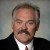# Why Is 1.5 Subtracted From the Short-term Estimate of Capability (Z.st) to Get the Long-term Estimate of Capability (Z.lt)?

ByDr. Mikel Harry

The capability of a process has two distinct but interrelated dimensions. First, there is short-term capability, or simply Z.st. Second, we have the dimension long-term capability, or just Z.lt. Finally, we note the contrast Z.shift = Z.st – Z.lt. By rearrangement, we assuredly recognize that Z.st = Z.lt + Z.shift and Z.lt = Z.st – Z.shift. So as to better understand the quantity Z.shift, we must consider some of the underlying mathematics.

The short-term (instantaneous) form of Z is given as Z.st = |SL – T| / S.st, where SL is the specification limit, T is the nominal specification and S.st is the short-term standard deviation. The short-term standard deviation would be computed as S.st = sqrt[SS.w / g(n – 1)], where SS.w is the sums-of-squares due to variation occurring within subgroups, g is the number of subgroups, and n is the number of observations within a subgroup.

It should be fairly apparent that Z.st assesses the ability of a process to repeat (or otherwise replicate) any given performance condition, at any arbitrary moment in time. Owing to the merits of a rational sampling strategy and given that SS.w captures only momentary influences of a transient and random nature, we are compelled to recognize that Z.st is a measure of instantaneous reproducibility. In other words, the sampling strategy must be designed such that Z.st does not capture or otherwise reflect temporal influences (time related sources of error). The metric Z.st must echo only pure error (random influences).

Now considering Z.lt, we understand that this metric is intended to expose how well the process can replicate a given performance condition over many cycles of the process. In its purest form, Z.lt is intended to capture and “pool” all of the observed instantaneous effects as well as the longitudinal influences. Thus, we compute Z.lt = |SL – M| / S.lt, where SL is the specification limit, M is the mean (average) and S.lt is the long-term standard deviation. The long-term standard deviation is given as S.lt = sqrt[SS.t / (ng – 1)], where SS.t is the total sums-of-squares. In this context, SS.t captures two sources of variation – errors that occur within subgroups (SS.w) as well as those that are created  between subgroups (SS.b). Given the absence of covariance, we are able to compute the quantity SS.t = SS.b + SS.w.

Handpicked Content:   Six Sigma Software Metrics, Part 4

In this context, we see that Z.lt provides a global sense of capability, not just a slice-in-time snapshot. Consequently, we recognize that Z.lt is time-sensitive, whereas Z.st is relatively independent of time. Based on this discussion, we can now better appreciate the contrast Z.st – Z.lt. This type of contrast poignantly underscores the extent to which time-related influences are able to unfavorably bias the instantaneous reproducibility of the process. Thus, we compute Z.shift = Z.st – Z.lt as a variable quantity that corrects, adjusts, or otherwise compensates the process capability for the influence of longitudinal effects.

If the contrast is related only to a comparison of short- and long-term random effects, the value of Z.shift can be theoretically established. For the common case ng = 30 and a type I decision error probability of .005, the equivalent mean shift will be approximately 1.5S.st. If the contrast also accounts for the occurrence of nonrandom effects, the equivalent mean shift cannot be theoretically established – it can only be empirically estimated or judgmentally asserted.